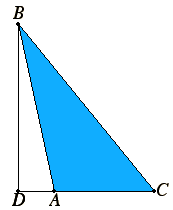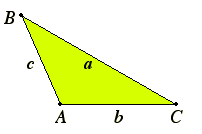# Proposition 12

In obtuse-angled triangles the square on the side opposite the obtuse angle is greater than the sum of the squares on the sides containing the obtuse angle by twice the rectangle contained by one of the sides about the obtuse angle, namely that on which the perpendicular falls, and the straight line cut off outside by the perpendicular towards the obtuse angle.
I.12

Let ABC be an obtuse-angled triangle having the angle BAC obtuse, and draw BD from the point B perpendicular to CA produced.

I say that the square on BC is greater than the sum of the squares on BA and AC by twice the rectangle CA by AD.II.4

Since the straight line CD has been cut at random at the point A, the square on DC equals the sum of the squares on CA and AD and twice the rectangle CA by AD.

Add the square on DB be added to each. Therefore the sum of the squares on CD and DB equals the sum of the squares on CA, AD, and DB plus twice the rectangle CA by AD.

I.47

But the square on CB equals the sum of the squares on CD and DB, for the angle at D is right, and the square on AB equals the sum of the squares on AD and DB, therefore the square on CB equals the sum of the squares on CA and AB plus twice the rectangle CA by AD, so that the square on CB is greater than the sum of the squares on CA and AB by twice the rectangle CA by AD.

Therefore in obtuse-angled triangles the square on the side opposite the obtuse angle is greater than the sum of the squares on the sides containing the obtuse angle by twice the rectangle contained by one of the sides about the obtuse angle, namely that on which the perpendicular falls, and the straight line cut off outside by the perpendicular towards the obtuse angle.

Q.E.D.

## Guide

This proposition for obtuse angles, together with the next one for acute triangles, complement the Pythagorean theorem, Proposition I.47, for right triangles. Prop.I.47 says that if triangle ABC has a right angle at A, then where a, b, and c are the sides opposite the angles A, B, and C, respectively.
 a2 = b2 + c2 In this proposition, II.12, the angle A is obtuse rather than right, and the conclusion is that a2 = b2 + c2 + 2c (AD) The next proposition, II.13 has a similar conclusion, but the hypothesis is that the angle at A is acute rather than obtuse.#### Law of Cosines

This conclusion is very close to the law of cosines for oblique triangles.

a2 = b2c2 – 2bc cos A,

since AD equals –b cos A, the cosine of an obtuse angle being negative. Trigonometry was developed some time after the Elements was written, and the negative numbers needed here (for the cosine of an obtuse angle) were not accepted until long after most of trigonometry was developed. Nonetheless, this proposition and the next may be considered geometric versions of the law of cosines.

Neither this nor the next is used in the rest of the Elements.